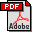Computing the Conditioning of the Components of a Linear Least Squares Solution
Marc Baboulin (University of Coimbra)
Jack Dongarra (University of Tennessee)
Serge Gratton (CNES)
Julien Langou (University of Colorado at Denver)
Abstract:
We address the accuracy of the results for the overdetermined full rank linear least squares problem. We recall theoretical results on conditioning of the least squares solution and the components of the solution when the matrix perturbations are measured in Frobenius or spectral norms. Then we define computable estimates for these condition numbers and we interpret them in terms of statistical quantities. In particular, we show that, in the classical linear statistical model, the ratio of the variance of one component of the solution by the variance of the right-hand side is exactly the condition number of this solution component when perturbations on the right-hand side are considered. We also provide fragment codes using LAPACK routines to compute the variance-covariance matrix and the least squares conditioning and we give the corresponding computational cost. Finally we present a small historical numerical example that was used by Laplace for computing the mass of Jupiter and experiments from the space industry with real physical data.
Keywords:
Numerical Algorithms for CS&EDownload the fullpaper
Toulouse | France | 2008 | June | 24  25  26  27